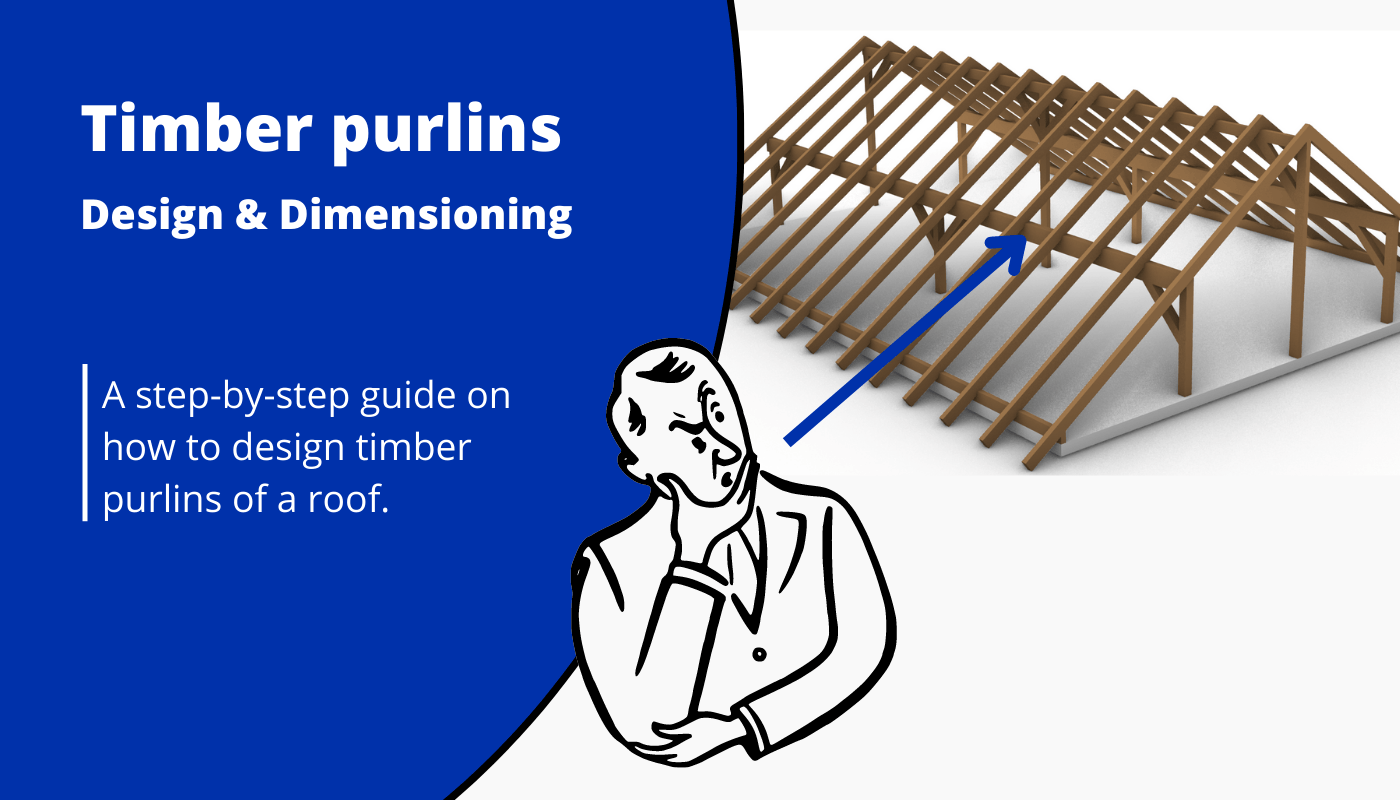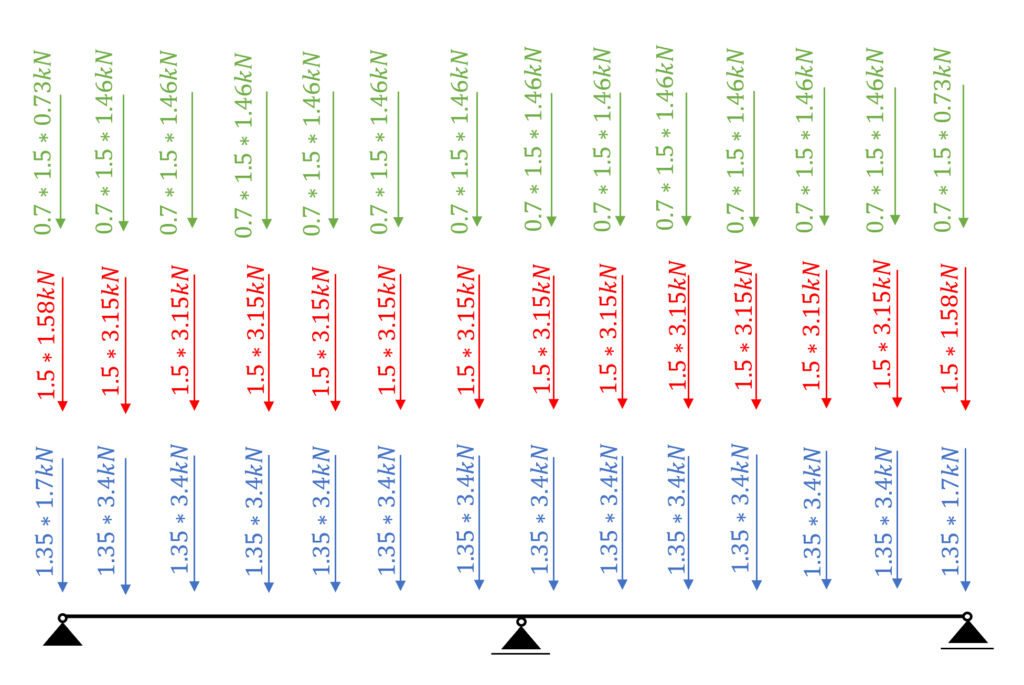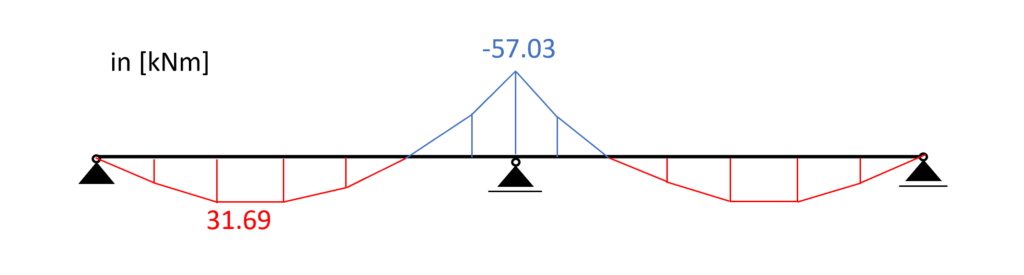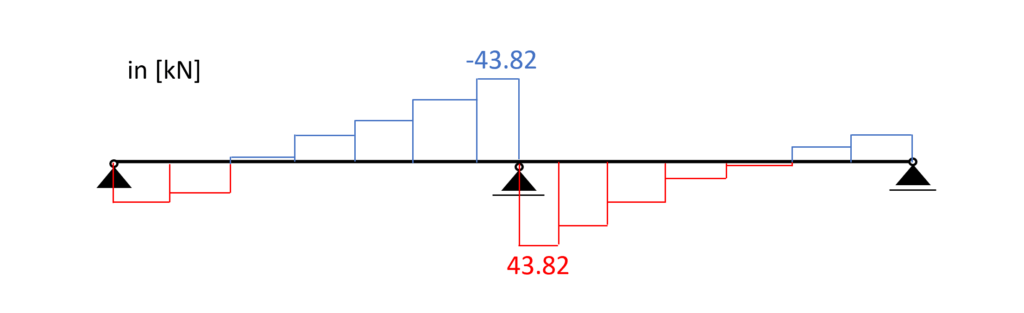# Timber Purlin roof design – Complete guideLast updated: June 7th, 2023

After we have designed the rafters of the purlin roof in the last article, we are now applying the support forces of the rafters to the purlins and design those elements….

Sounds exciting, right?🔥

Let’s get into it👍.

To be on the same page, we should firstly describe what a purlin roof is, and what are the purlins of it? 🤔

I know all this sounds quite complicated, but don’t worry, we will explain it practically with examples and pictures.

Let me explain to you the steps we have to do to dimension a rafter. Let’s have a look at the steps we need to follow.

1. Choose a right statical system, continuous beam in our case
4. Choose a timber material and find material properties ($k_{mod}$, $f_{c.0.k}$, $f_{m.k}$, $\gamma_{M}$)
5. Assume the width w and height h of the cross section
6. Verify the beam for bending. If not verified increase the width or height of the beam and do the calculation again
7. Verify the beam for shear. If not verified increase the width or height of the beam and do the calculation again
8. Verify the beam for the instantaneous deflection criteria. If not verified increase the width or height of the beam and do the calculation again
9. Verify the beam for the final deflection criteria. If not verified increase the width or height of the beam and do the calculation again
10. If all of those checks are now verified, then you have figured out the correct dimensions of the beam

## Statical system

In general the statical system of the purlin is a beam. Depending on how many support it has either a simply supported or a continous beam. In our case we have a continuous beam with 3 supports.

To not loose the connection to the overall roof when we are talking about the purlin beam we mean the red marked element of the timber roof.

.. and the supports (roller and pin) are the columns.

As we promised in the last article the support forces of the rafters are applied as point loads on the purlin roof – in the opposite direction!

Therefore we need to calculate the support forces of the rafters due to the characteristic loads. Another way is to calculate the biggest design support loads (from load combinations). We are choosing the first way.

The characteristic loads will not be derived in this article. We explained the calculation of dead, live, wind and snow loads for pitched roofs thoroughly in previous articles.

As already mentioned we are interested in the characteristic support forces of the rafters which we then apply to the purlin.

And for 14 rafters we get 14 point loads 😁

Let’s do an example. We will apply the characteristic snowload to the rafter to see what pointload needs to get applied to the purlins.

.. When designing the “middle” purlin, we can now apply 1.46 kN to the purlin beam as the characteristic snow load.

Now we do this for all the characteristic loads we have. To speed things a bit up we are only considering the worst-case wind load value.

3D FE programmes can take all the different values into account. This would be too much data in our “simple” tutorial.

The self-weight of the purlin has to be added to $g_{k}$ as well.

When you design an element like the purlin, you do not know the exact dimensions of the cross-section. Therefore, you assume the width and height.

Once the correct cross-section is found, the self-weight gets updated as well.

However, we will not do this in this tutorial because it makes things more complicated and the focus of this tutorial is on the design of the timber elements.

Luckily we have already written an extensive article about what load combinations are and how we use them. In case you need to brush up on it you can read the blog post here.

❗We are choosing to include $w_{k.H.}$ = 0.72 kN as the wind load in the load combinations and the following calculations to keep it clean.

In principle, you should consider all load combinations. However, with a bit more experience, you might be able to exclude some of the values.

In modern FE programs, multiple values for the wind load can be applied, and load combinations automatically generated.

So the computer is helping us a lot. Just keep in mind that you should include all wind loads, but because of simplicity we do only consider 1 value in this article😁.

## Rafter timber material

For this blog post/tutorial, we are choosing a Glulam GL 30h. More comments on which timber material to pick and where to get the properties from were made here.

The following characteristic strength and stiffness parameters were found online from a manufacturer.

## Modification factor $k_{mod}$

If you do not know what the modification factor $k_{mod}$ is, we wrote an explanation to it in a previous article, which you can check out.

Since we want to keep everything as short as possible, we are not going to repeat it in this article – we are only defining the values of $k_{mod}$.

For a residential house which is classified as Service class 1 according to EN 1995-1-1 2.3.1.3 we extract the following load durations for the different loads.

From EN 1995-1-1 Table 3.1 we get the $k_{mod} values for the load durations and a Glulam GL 30h (Glued laminated timber). ## Partial factor$\gamma_{M}$According to EN 1995-1-1 Table 2.3 the partial factor$\gamma_{M}$is defined as$\gamma_{M} = 1.3$## Assumption of width and height of rafter We are defining the width w and height h of the C24 structural wood Cross-section as Width w = 200 mm Height h = 320 mm Those values are based on the experience of the designer. 💡We highly recommend doing any calculation in a program where you can always update values and not by hand on a piece of paper! I made that mistake in my bachelor. In any course and even in my bachelor thesis, I calculated everything except the forces (FE program) on a piece of paper. Now that we know the width and the height of the Cross-section we can calculate the Moment of inertias$I_{y}$and$I_{z}$.$I_{y} = \frac{w * h^3}{12} = \frac{200mm * (320mm)^3}{12} = 5.46 * 10^8 mm^4 I_{z} = \frac{w^3 * h}{12} = \frac{(200mm)^3 * 320mm}{12} = 2.13 * 10^8 mm^4 $## ULS Design In the ULS (ultimate limit state) Design we verify the stresses in the timber members due to bending and shear. In order to calculate the stresses of our purlins, we need to calculate the Bending Moments and shear forces due to different loads. This is a little bit more work than for a flat roof/beam (simply supported) because our statical system has 3 supports (statically indeterminate) and we are having point loads. ### Calculation of bending moment and shear forces We use a FE program to calculate the bending moments and shear forces. Load combination 5 with live load as leading, snow and wind load as reduced load leads to the highest results however due to different$k_{mod}$values for LC5 (instantaneous) and LC3 (medium), LC3 will lead to the highest utilization$\eta$. #### Load combination 3Load combination 3 | Dead load, Live load, Snow load #### Load combination 3 – Bending momentsBending moments | Purlin | Load combination 3 #### Load combination 3 – Shear forcesShear forces | Purlin | Load combination 3 ### Bending From the max. bending moment (midsupport: -57.03 kNm) we can calculate the stress in the most critical cross section. Bending stress:$\sigma_{m} = \frac{M_{d}}{I_{y}} * \frac{h}{2} = \frac{57.03 kNm}{5.46*10^{-4}m^4} * \frac{0.32m}{2} = 16.7 MPa$Resistance stresses of the timber material:$ f_{d} = k_{mod} * \frac{f_{k}}{\gamma_{m}} $##### Utilization according to EN 1995-1-1 (6.19)$\eta = \frac{\sigma_{m}}{f_{m.d}} = 0.905 < 1.0$### Shear From the max. shear force (midsupport: 43.82 kN) we can calculate the shear stress in the most critical cross section. Shear stress:$\tau_{d} = \frac{3*V}{2*w*h} =  \frac{3*43.82 kN}{2*0.2m*0.32m} = 1.03 MPa$Resistance stresses of the timber material:$ f_{v} = k_{mod.M} * \frac{f_{v}}{\gamma_{m}}  f_{v} = 0.8 * \frac{3.5 MPa}{1.3} = 2.15 MPa$##### Utilization according to EN 1995-1-1 (6.13)$\eta = \frac{\tau_{v}}{f_{v}} = 0.477 < 1.0$## SLS Design We also discussed the SLS design a bit more in detail in a previous article. In this blog post we are not explaining too much but rather show the calculations😊. ### Instantaneous deformation$u_{inst}u_{inst}$(instantaneous deformation) of our beam can be calculated with the load of the characteristic load combination. As for the bending moments, shear and axial forces, we are using a FE programme to calculate the deflections due to our Load combinations. LC 5 of the characteristic SLS load combinations leads to the largest deflection u.$u_{inst}$= 11.7 mm Unfortunately, EN 1995-1-1 Table 7.2 recommends values for$w_{inst} only for “Beams on two supports” and “Cantilevering beams” and not for a continuous beam, which is our case.

However, the limits of the deflection can be agreed upon with the client and the structure is not collapsing due to too large deflections if the rafter is verified for all ULS calculations.

So for the sake of this tutorial we are using the value for “Beam on two supports” from EN 1995-1-1 Table 7.2.

$w_{inst}$ = l/300 = 6.5m/300 = 21.7 mm

##### Utilization

$\eta = \frac{u_{inst}}{w_{inst}} = \frac{11.7mm}{21.7mm} = 0.54$

### Final deformation $u_{fin}$

$u_{fin}$ (final deformation) of our beam can be calculated by adding the creep deformation $u_{creep}$ to the instantaneous deflection $u_{inst}$.

Therefore, we will calculate the creep deflection with a FE program.

This might be a bit quick, but we have already covered the basics in the article about the timber beam dimensioning.

So check that out if you want to know exactly how to calculate $u_{creep}$ by hand.

$u_{creep}$ = 3.36mm

Adding the creep to the instantaneous deflection leads to the final deflection.

$u_{fin} = u_{inst} + u_{creep} = 11.7mm + 3.36mm= 15.06mm$

Limit of $u_{fin}$ according to EN 1995-1-1 Table 7.2

$w_{fin}$ = l/150 = 6.5m/150 = 43.3 mm

##### Utilization

$\eta = \frac{u_{fin}}{w_{fin}} = \frac{15.06mm}{43.3mm} = 0.35$

🎉 Finishing off with the final deflection criteria, the purlin is now designed and verified.

The next step is to apply the support forces to the columns or walls – depending on how the design looks like – and design the columns. So see you in the next tutorial😎.